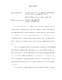## A STUDY OF EFFECTIVE THEORY APPROACHES TO PROBLEMS IN NUCLEAR PHYSICS2010
##### Authors
Chen, Panying
The effective field theory is an efficient tool in dealing with physics involving separate scales. A typical effective field theory approach involves calculating the matching conditions for the desired parameters at some cutoff scale and obtain the renormalization group equation for the effective theory. In this thesis we study a collection of effective theories including the non-relativistic QED, the chiral perturbation theory, the soft-collinear effective theory, and their applications in nuclear physics. We study the angular momentum operator in the non-relativistic QED (NRQED). We construct its gauge-invariant decomposition into spin and orbital angular momentum operators of electrons and photons and calculate the matching conditions of operators between QED and NRQED up to one loop. We perform the matching with both dimensional regularization and UV cutoff $\Lambda$. We apply the result in the Hydrogen-like system and calculate the radiation correction of the orbital angular momentum. We study the CP-violating operators in chiral perturbation theory. We apply our general, model-independent result onto the left-right symmetric model and relate the desired operator to the standard model penguin operators through $SU(3)_L$ &times $SU(3)_R$ chiral symmetry. We use the lattice result for the standard model and acquire a more strict lower bound for the mass of right-hand boson in LRSM. We study the polarized gluon distribution $\Delta g(x)$ in a longitudinally polarized proton. The first result from the MIT bag model as well as the non-relativistic quark model shows that $\Delta g(x)$ is positive at all $x$. The total gluon helicity $\Delta G$ from the bag model is about $0.3\hbar$ at the scale of 1 GeV, considerably smaller than previous theoretical expectations. We study deep-inelastic scattering factorization on a nucleon in the end-point regime $x_B \sim 1-{\cal O}( \Lambda_{\rm QCD}/Q)$ with an approach in soft-collinear effective theory. Refactorization of the scale $(1-x_B)Q^2$ in the coefficient function can be made in the SCET and remains valid in the end-point regime. On the other hand, the traditional refactorization approach introduces the spurious scale $(1-x_B)Q$ in various factors, which drives them nonperturbative in the region of our interest. We show how to improve the situation by introducing a rapidity cut-off scheme, and how to recover the effective theory refactorization by choosing appropriately the cut-off parameter. Through a one-loop calculation, we demonstrate explicitly that the proper soft subtractions must be made in the collinear matrix elements to avoid double counting.